# Lathyrus vernus IPMs

In this vignette, we will use the lathyrus dataset to illustrate the estimation of integral projection models (IPMs). To reduce file size, we have prevented some statements from running if they produce long stretches of output. Examples include most summary() calls - to run these statements, eliminate the leading hashtags and then run them. We have also written this vignette using the default Ehrlén format for all historical MPMs. All examples can be run in deVries format as well. This vignette is only a sample analysis. Detailed information and instructions on using lefko3 are available through a free online e-book called lefko3: a gentle introduction, available on the projects page of the Shefferson lab website.

### ORGANISM AND POPULATION

Lathyrus vernus (family Fabaceae) is a long-lived forest herb, native to Europe and large parts of northern Asia. Please see our description of the plant, study site, and methods in our vignette on raw ahistorical MPM creation and analysis.

### BASIC WORKFLOW

The dataset that we have provided is organized in horizontal format, meaning that each row holds all of the data for a single, unique individual, and columns correspond to individual condition in particular monitoring occasions (which we refer to as years here, since there was one main census in each year). The original spreadsheet file used to keep the dataset has a repeating pattern to these columns, with each year having a similarly arranged group of variables. Let’s load the dataset.

data(lathyrus)
dim(lathyrus)
#>  1119   38
#summary(lathyrus)

This dataset includes information on 1,119 individuals arranged horizontally, so there are 1,119 rows with data. There are 38 columns. The first two columns are variables giving identifying information about each individual. This is followed by four sets of nine columns, each named VolumeXX, lnVolXX, FCODEXX, FlowXX, IntactseedXX, Dead19XX, DormantXX, Missing19XX, and SeedlingXX, where XX corresponds to the year of observation and with years organized consecutively. Thus, columns 3-11 refer to year 1988, columns 12-20 refer to year 1989, etc. This strictly repeated pattern allows us to manipulate the original dataset quickly and efficiently via lefko3. There are four years of data, from 1988 to 1991. Ideally, we should also have arranged the columns in the same order for each year, with years in consecutive order with no extra columns between them. This order is not required, provided that we input all variable names in correct order when transforming the dataset later.

#### Step 1. Life history model development

To begin, we will create a stageframe. A stageframe is a data frame that describes all stages in the life history of the organism, in a way usable by the functions in this package and using stage names and classifications that completely match those used in the dataset. It must include complete descriptions of all stages that occur in the dataset, with each stage defined uniquely. Since this object can be used for automated classification of individuals, all sizes, reproductive states, and other characteristics defining each stage in the dataset need to be accounted for explicitly. This can be difficult if a few data points do not fit neatly into any stge description, so great care must be taken to include all relevant size values and values of other descriptor variables occurring within the dataset. The final description of each stage occurring in the dataset must not completely overlap with any other stage, although partial overlap is allowed. We will base our stageframe on the life history model provided in Ehrlén (2000), but use a different size classification based on leaf volume to allow IPM construction and make all mature stages other than vegetative dormancy reproductive, as shown in Figure 6.1.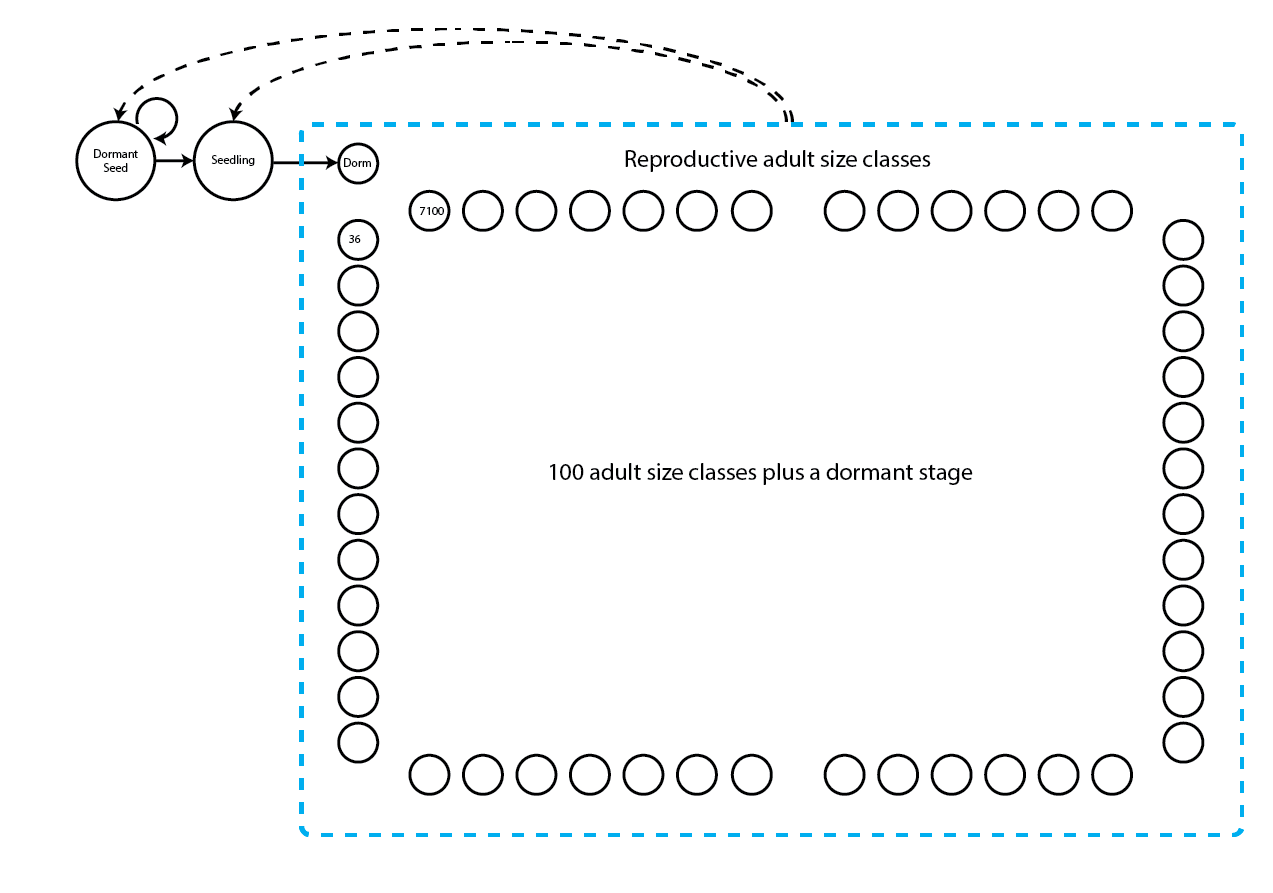Figure 6.1. Life history model of Lathyrus vernus. Not all adult classes are shown. Survival transitions are indicated with solid arrows, while fecundity transitions are indicated with dashed arrows.

In the stageframe code below, we show that we want an IPM by choosing two stages that serve as the size limits for IPM size classification. These two size classes should have exactly the same characteristics in the stageframe other than size. By choosing these two size limits, we can skip adding and describing the many size classes that will fall between these limits - function sf_create() will create all of these for us. We mark these limits in the vector that we load into the stagenames option using the string ipm. Package lefko3 will then create and name all IPM size classes according to its own conventions. The default number of size classes is 100 bins, which can be changed using the ipmbins option.

sizevector <- c(0, 100, 0, 1, 7100)
stagevector <- c("Sd", "Sdl", "Dorm", "ipm", "ipm")
repvector <- c(0, 0, 0, 1, 1)
obsvector <- c(0, 1, 0, 1, 1)
matvector <- c(0, 0, 1, 1, 1)
immvector <- c(1, 1, 0, 0, 0)
propvector <- c(1, 0, 0, 0, 0)
indataset <- c(0, 1, 1, 1, 1)
binvec <- c(0, 100, 0.5, 1, 1)
lathframeipm <- sf_create(sizes = sizevector, stagenames = stagevector,
repstatus = repvector, obsstatus = obsvector, propstatus = propvector,
indataset = indataset, binhalfwidth = binvec, ipmbins = 100, roundsize = 3)
#lathframeipm

This stageframe has 103 stages. The IPM portion technically starts with the fourth stage and keeps going through the 103rd stage. Stage names within this range are concatenations of the size centroid (designated with sz), and, given a limited string length, the reproductive status, maturity status, and observation status. The first three stages, which fall outside of the IPM classification, are left unaltered. Note that we are using the midpoint approach to determining the size bins here, using the default bin halfwidths of 0.5 (since all size bins are using this default, we do not include a binhalfwidth vector option in the sf_create() input). However, we could have used the sizemin and sizemax options to more deliberately set the size bin minima and maxima instead, although that would have been a more tedious approach in a high dimensonal object like an IPM.

#### Step 2a. Dataset standardization

To work with this dataset, we first need to format the data into vertical format, in which each row corresponds to the state of a single individual in two (if ahistorical) or three (if historical) consecutive time intervals. In the input to verticalize3() below, we utilize a repeating pattern of variable names arranged in the same order for each monitoring occasion. This arrangement allows us to enter only the first variable in each set, as long as noyears and blocksize are set properly and no gaps or shuffles appear in the dataset. The data management functions in lefko3 do not require such repeating patterns, but they do make the required input in the function shorter and more succinct. Because this is an IPM, we will need to estimate linear models of vital rates. This will require us to avoid NAs in size and fecundity (fortunately, in this dataset, NA is equivalent to 0), so we will set NAas0 = TRUE to inform R to treat NAs as zeroes. We will also set NRasRep = TRUE because we will assume that all adult stages other than dormancy are reproductive, and there are mature individuals in the dataset that do not reproduce but need to be included in reproductive stages.

Note that prior to standardizing the dataset, we will create a new variable to code individual identity, since different plants in different subpopulations use the same identifiers. To see a more detailed summary of the standardized dataset, please remove full = FALSE from the summary_hfv() call.

lathyrus$indiv_id <- paste(lathyrus$SUBPLOT, lathyrus$GENET) lathvertipm <- verticalize3(lathyrus, noyears = 4, firstyear = 1988, individcol = "indiv_id", blocksize = 9, juvcol = "Seedling1988", sizeacol = "Volume88", repstracol = "FCODE88", fecacol = "Intactseed88", deadacol = "Dead1988", nonobsacol = "Dormant1988", stageassign = lathframeipm, stagesize = "sizea", censorcol = "Missing1988", censorkeep = NA, censorRepeat = TRUE, censor = TRUE, NAas0 = TRUE, NRasRep = TRUE) summary_hfv(lathvertipm, full = FALSE) #> #> This hfv dataset contains 2527 rows, 54 variables, 1 population, #> 1 patch, 1053 individuals, and 3 time steps. Before we move on to the next steps in analysis, let’s take a closer look at fecundity. In this dataset, fecundity is mostly a count of intact seeds, and only differs in six cases where the seed output was estimated based on other models. To see this, try the following code, which focuses on fecundity in time t. # Length of fecundity variable in t: length(lathvertipm$feca2)
#>  2527
# Number of non-integer entries:
length(which(lathvertipm$feca2 != round(lathvertipm$feca2)))
#>  6

We see that we have quite a bit of fecundity data, and that it is overwhelmingly but not exclusively integer. So, we can either treat fecundity as a continuous variable, or round the values and treat fecundity as a count variable. We will choose the latter approach in this analysis.

lathvertipm$feca3 <- round(lathvertipm$feca3)
lathvertipm$feca2 <- round(lathvertipm$feca2)
lathvertipm$feca1 <- round(lathvertipm$feca1)

Although we wish to treat fecundity as a count, it is still not clear what underlying distribution we should use. The Poisson distribution assumes that the mean and variance are equal, and so we can test this assumption using a chi-squared test. If it is not significantly different, then we may use some variant of the Poisson distribution. If the data are significantly over-dispersed, then we should use the negative binomial distribution. If fecundity of 0 is possible in reproductive stages, as in cases where reproductive status is defined by flowering rather than by offspring production, then we should also test whether the number of zeroes is significantly greater than expected under these distributions, and use a zero-inflated distribution if so.

Let’s formally test our assumptions. We will use the hfv_qc() function, which will allow us to assess the quality of the data from the viewpoint of the linear models to be built later. We will use most of the input from the modelsearch() call that we will conduct later.

hfv_qc(data = lathvertipm, vitalrates = c("surv", "obs", "size", "fec"),
juvestimate = "Sdl", indiv = "individ", year = "year2", age = "obsage")
#> Survival:
#>
#>   Data subset has 54 variables and 2246 transitions.
#>
#>   Variable alive3 has 0 missing values.
#>   Variable alive3 is a binomial variable.
#>
#>
#> Observation status:
#>
#>   Data subset has 54 variables and 2121 transitions.
#>
#>   Variable obsstatus3 has 0 missing values.
#>   Variable obsstatus3 is a binomial variable.
#>
#>
#> Primary size:
#>
#>   Data subset has 54 variables and 1916 transitions.
#>
#>   Variable sizea3 has 0 missing values.
#>   Variable sizea3 appears to be a floating point variable.
#>   1256 elements are not integers.
#>   The minimum value of sizea3 is 3.4 and the maximum is 6646.
#>   The mean value of sizea3 is 512.8 and the variance is 507200.
#>   The value of the Shapiro-Wilk test of normality is 0.7134 with P = 3.014e-49.
#>   Variable sizea3 differs significantly from a Gaussian distribution.
#>
#>   Variable sizea3 is fully positive, lacking even 0s.
#>
#>
#> Fecundity:
#>
#>   Data subset has 54 variables and 2246 transitions.
#>
#>   Variable feca2 has 0 missing values.
#>   Variable feca2 appears to be an integer variable.
#>
#>   Variable feca2 is fully non-negative.
#>
#>   Overdispersion test:
#>     Mean feca2 is 1.282
#>     The variance in feca2 is 23.21
#>     The probability of this dispersion level by chance assuming that
#>     the true mean feca2 = variance in feca2,
#>     and an alternative hypothesis of overdispersion, is 0
#>     Variable feca2 is significantly overdispersed.
#>
#>   Zero-inflation and truncation tests:
#>     Mean lambda in feca2 is 0.2774
#>     The actual number of 0s in feca2 is 1980
#>     The expected number of 0s in feca2 under the null hypothesis is 623
#>     The probability of this deviation in 0s from expectation by chance is 0
#>     Variable feca2 is significantly zero-inflated.
#>
#>
#> Juvenile survival:
#>
#>   Data subset has 54 variables and 281 transitions.
#>
#>   Variable alive3 has 0 missing values.
#>   Variable alive3 is a binomial variable.
#>
#>
#> Juvenile observation status:
#>
#>   Data subset has 54 variables and 210 transitions.
#>
#>   Variable obsstatus3 has 0 missing values.
#>   Variable obsstatus3 is a binomial variable.
#>
#>
#> Juvenile primary size:
#>
#>   Data subset has 54 variables and 193 transitions.
#>
#>   Variable sizea3 has 0 missing values.
#>   Variable sizea3 appears to be a floating point variable.
#>   127 elements are not integers.
#>   The minimum value of sizea3 is 2.1 and the maximum is 61.
#>   The mean value of sizea3 is 11.23 and the variance is 50.81.
#>   The value of the Shapiro-Wilk test of normality is 0.5997 with P = 5.72e-21.
#>   Variable sizea3 differs significantly from a Gaussian distribution.
#>
#>   Variable sizea3 is fully positive, lacking even 0s.
#>
#>
#> Juvenile maturity status:
#>
#>   Data subset has 54 variables and 210 transitions.
#>
#>   Variable matstatus3 has 0 missing values.
#>   Variable matstatus3 is a binomial variable.

All of the probability variables look like binomials, so we have no problem there. Size and juvenile size appear to be continuous variables that differ significantly from the assumptions of the Gaussian distribution. However, we will still use a Gaussian distribution in this case, for instructional purposes. Fecundity is a count variable with significant overdispersion and significantly more zeros than expected, so we will use a zero-inflated negative binomial distribution.

#### Step 2b: Develop supplemental information for matrix estimation

Now we will create supplement tables, which provide extra data for matrix estimation that is not included in the main demographic dataset. Specifically, we will provide the seed dormancy probability and germination rate, which are given as transitions from the dormant seed stage to another year of seed dormancy or to the germinated seedling stage, respectively. We assume that the germination rate is the same regardless of whether seed was produced in the previous year or has been in the seedbank for longer. We will incorporate these terms both as fixed constants for specific transitions within the resulting matrices, and as multipliers for fecundity, since ultimately fecundity will be estimated as the production of seed multiplied by the seed germination rate or the seed dormancy rate. The fecundity multipliers will also serve to tell R which transitions are the fecundity transitions. Because some individuals stay in the seedling stage for only 1 year, and the seed stage itself cannot be observed and so does not exist in the dataset, we will also set a proxy set of transitions assuming that transitions from seed in occasion t-1 to seedling in occasion t to all mature stages in occasion t+1 are equal to the equivalent transitions from seedling in both occasions t-1 and t. We will start with the ahistorical case, and then move on to the historical case, where we also need to input the corresponding stages in occasion t-1 and transition types from occasion t-1 to t for each transition.

lathsupp2 <- supplemental(stage3 = c("Sd", "Sdl", "Sd", "Sdl"),
stage2 = c("Sd", "Sd", "rep", "rep"),
givenrate = c(0.345, 0.054, NA, NA),
multiplier = c(NA, NA, 0.345, 0.054),
type = c(1, 1, 3, 3), stageframe = lathframeipm, historical = FALSE)

lathsupp3 <- supplemental(stage3 = c("Sd", "Sd", "Sdl", "Sdl", "npr", "Sd", "Sdl"),
stage2 = c("Sd", "Sd", "Sd", "Sd", "Sdl", "rep", "rep"),
stage1 = c("Sd", "rep", "Sd", "rep", "Sd", "mat", "mat"),
eststage3 = c(NA, NA, NA, NA, "npr", NA, NA),
eststage2 = c(NA, NA, NA, NA, "Sdl", NA, NA),
eststage1 = c(NA, NA, NA, NA, "Sdl", NA, NA),
givenrate = c(0.345, 0.345, 0.054, 0.054, NA, NA, NA),
multiplier = c(NA, NA, NA, NA, NA, 0.345, 0.054),
type = c(1, 1, 1, 1, 1, 3, 3), type_t12 = c(1, 2, 1, 2, 1, 1, 1),
stageframe = lathframeipm, historical = TRUE)

#lathsupp2
#lathsupp3

#### Step 3. Tests of history, and vital rate modeling

Integral projection models (IPMs) require functions of vital rates to populate them. Here, we will develop these functions as linear models using modelsearch(). This function automates several crucial and complex tasks in MPM analysis. Specifically, it automates 1) the building of global models for each vital rate requested, 2) the exhaustive construction of all reduced models, and 3) the selection of the best-fit models. This function also allows us to test whether individual history affects demography. Setting historical = TRUE fits size and/or reproductive status in occasions t and t-1 into global models, while setting historical = FALSE limits testing to the impacts of state in occasion t only. The model building and selection protocols can then be used to see if history has a significant impact on a vital rate, by assessing whether a historical term is retained in the best-fit model.

First, we will create the historical models to assess whether history is a significant influence on vital rates.

lathmodels3ipm <- modelsearch(lathvertipm, historical = TRUE, approach = "mixed",
suite = "main", vitalrates = c("surv", "obs", "size", "fec"),
juvestimate = "Sdl", bestfit = "AICc&k", sizedist = "gaussian",
fecdist = "negbin", fec.zero = TRUE, indiv = "individ", year = "year2",
year.as.random = TRUE, juvsize = TRUE, quiet = "partial")
#>
#> Developing global model of survival probability...
#>
#> Global model of survival probability developed. Proceeding with model dredge...
#>
#> Developing global model of observation probability...
#>
#> Global model of observation probability developed. Proceeding with model dredge...
#>
#> Developing global model of primary size...
#>
#> Global model of primary size developed. Proceeding with model dredge...
#>
#> Reproduction probability will be treated as constant.
#>
#> Developing global model of fecundity...
#>
#> Global model of fecundity developed. Proceeding with model dredge...
#>
#> Developing global model of juvenile survival probability...
#>
#> Global model of juvenile survival probability developed. Proceeding with model dredge...
#> Warning: Juvenile maturity status in time t+1 appears to be constant, and so will be set to constant.
#>
#> Developing global model of juvenile observation probability...
#>
#> Global model of juvenile observation probability developed. Proceeding with model dredge...
#>
#> Developing global model of juvenile primary size...
#>
#> Could not properly estimate a global model for juvenile size.
#> Warning: Juvenile reproductive status in time t+1 appears to be constant, and so will be set to constant.
#>
#> Finished selecting best-fit models.
#summary(lathmodels3ipm)

We see here, as before, that status in occasion t-1 exerts an influence on some vital rates, particularly survival to occasion t+1, size in occasion t+1, and fecundity. So, the historical IPM is the correct choice here. Accuracy is also quite high for adult survival and juvenile and adult observation, but quite poor for primary size and fecundity.

We will also create an ahistorical IPM for comparison. For that purpose, we will create the ahistorical linear model set.

lathmodels2ipm <- modelsearch(lathvertipm, historical = FALSE,
approach = "mixed", suite = "main",
vitalrates = c("surv", "obs", "size", "fec"), juvestimate = "Sdl",
bestfit = "AICc&k", sizedist = "gaussian", fecdist = "negbin",
fec.zero = TRUE, indiv = "individ", year = "year2", year.as.random = TRUE,
juvsize = TRUE, quiet = "partial")
#>
#> Developing global model of survival probability...
#>
#> Global model of survival probability developed. Proceeding with model dredge...
#>
#> Developing global model of observation probability...
#>
#> Global model of observation probability developed. Proceeding with model dredge...
#>
#> Developing global model of primary size...
#>
#> Global model of primary size developed. Proceeding with model dredge...
#>
#> Reproduction probability will be treated as constant.
#>
#> Developing global model of fecundity...
#>
#> Global model of fecundity developed. Proceeding with model dredge...
#>
#> Developing global model of juvenile survival probability...
#>
#> Global model of juvenile survival probability developed. Proceeding with model dredge...
#> Warning: Juvenile maturity status in time t+1 appears to be constant, and so will be set to constant.
#>
#> Developing global model of juvenile observation probability...
#>
#> Global model of juvenile observation probability developed. Proceeding with model dredge...
#>
#> Developing global model of juvenile primary size...
#>
#> Could not properly estimate a global model for juvenile size.
#> Warning: Juvenile reproductive status in time t+1 appears to be constant, and so will be set to constant.
#>
#> Finished selecting best-fit models.
#summary(lathmodels2ipm)

#### Step 4. IPM estimation

We will now create the historical suite of matrices covering the years of study. These matrices will be extremely large, but they are sparse and we will build them in sparse format, so they should not take too much memory.

lathmat3ipm <- flefko3(stageframe = lathframeipm, modelsuite = lathmodels3ipm,
supplement = lathsupp3, data = lathvertipm, reduce = FALSE,
sparse_output = TRUE)
#summary(lathmat3ipm)

These are giant matrices. With 10,609 rows and columns, there are a total of 112,550,881 elements per matrix. But they are also amazingly sparse - with about 960,205 elements estimated per matrix, only 0.9% of elements per matrix are non-zero. The survival probability sums all look good, so we appear to have no problems with overly large given and proxy survival transitions provided through our supplemental tables. Let’s now build the ahistorical IPMs.

lathmat2ipm <- flefko2(stageframe = lathframeipm, modelsuite = lathmodels2ipm,
supplement = lathsupp2, data = lathvertipm, reduce = FALSE)
#summary(lathmat2ipm)

The ahistorical IPMs are certainly smaller than the historical IPMs, but are nonetheless quiet large. Although huge, these matrices are not sparse - an average of 9,180.3 elements out of 10,609 per matrix are estimated (86.5%; note that the summary() function only counts elements as estimated if they equal a value other than 0, leading the exact number of estimated elements to vary among matrices when elements are estimated at near 0).

Let’s do a further comparison - let’s view a matrix plot of each kind of MPM, ahistorical and then historical. First, the ahistorical plot.

image3(lathmat2ipm, used = 1)
#> []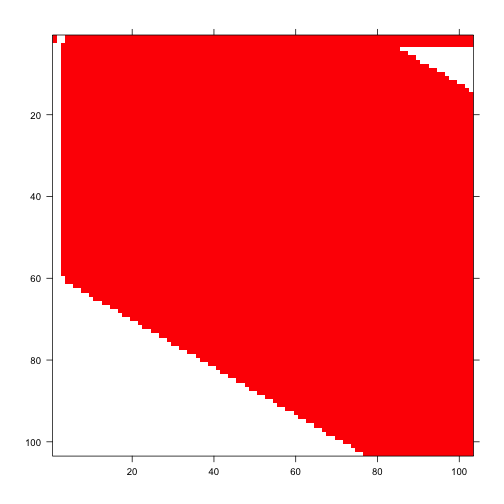Figure 6.2. Image of ahistorical projection matrix

Now the historical plot.

image3(lathmat3ipm, used = 1)
#> []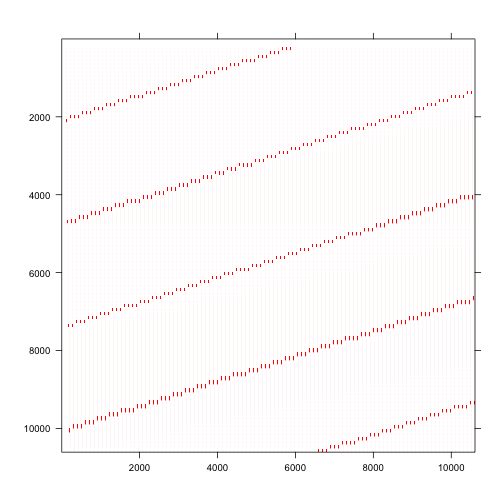Figure 6.3. Image of historical projection matrix

The plots above show that the ahistorical matrix is large but dense, mostly full of non-zero entries (the colored elements correspond to non-zero elements). In contrast, the historical matrix is huge and sparse, mostly full of zeroes with a general pattern to the distribution of non-zero elements.

Now let’s estimate the mean IPM matrices. We will estimate one mean matrix each, because we did not separate patches in the data reorganization and vital rate modeling. As a check, let’s also look over the summaries.

lath2ipmmean <- lmean(lathmat2ipm)
lath3ipmmean <- lmean(lathmat3ipm)
#summary(lath2ipmmean)
#summary(lath3ipmmean)

All looks fine! Let’s also take a look at a portion of one of the conditional historical matrices, particularly the matrix conditional on vegetative dormancy in occasion t-1. This matrix can be compared to the ahistorical mean to assess the impacts of history on the matrix elements themselves. Please remember to remove the hashtag.

l3mcond <- cond_hmpm(lath3ipmmean)
#l3mcond$Mcond[]$Dorm

#### Step 5. MPM analysis

Now let’s estimate and plot the deterministic population growth rate, $$\lambda$$, for each year. We find that the ahistorical and historical estimates of $$\lambda$$ are roughly in line with each other.

ipm2lambda <- lambda3(lathmat2ipm)
ipm3lambda <- lambda3(lathmat3ipm)

plot(lambda ~ year2, data = ipm2lambda, xlab = "Year", ylab = "Lambda",
ylim = c(0.65, 1.00), type = "l", lwd = 2, bty = "n")
lines(lambda ~ year2, data = ipm3lambda, lwd = 2, lty = 2, col = "red")
legend("bottomleft", c("ahistorical", "historical"), lty = c(1, 2),
col = c("black", "red"), lwd = 2, bty = "n")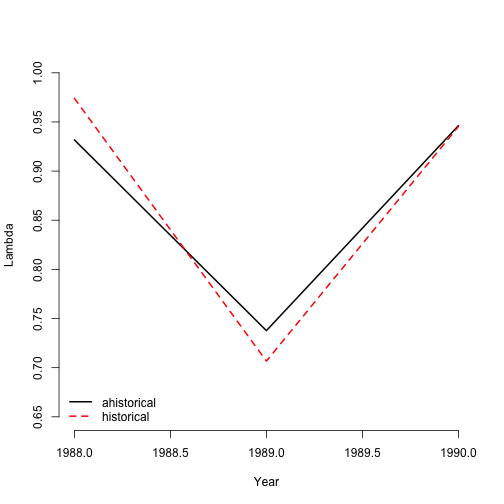Figure 6.4. Ahistorical vs. historical lambda

Let’s now look at $$\lambda$$ for the mean matrices and compare with the stochastic growth rate, $$a = \text{log} \lambda _{S}$$. We will set the number of simulations low in the historical case in order to keep the amount of memory used and computation time low, because the size of the historical matrices will use up plenty of both (the default is 10,000). We will also set the seed for the random number generator to make our results reproducible.

lambda3(lath2ipmmean)
#>   pop patch    lambda
#> 1   1  <NA> 0.8858591
lambda3(lath3ipmmean)
#>   pop patch   lambda
#> 1   1  <NA> 0.902619

set.seed(42)
slambda3(lathmat2ipm)
#>   pop patch          a         var         sd           se
#> 1   1  <NA> -0.1252965 0.006844859 0.08273366 0.0008273366
set.seed(42)
slambda3(lathmat3ipm, times = 1000)
#>   pop patch          a       var         sd          se
#> 1   1  <NA> -0.1077984 0.0083111 0.09116523 0.002882898

The historical growth rate is larger than the ahistorical in both deterministic and stochastic analyses, although all four numbers suggest a declining population. Now let’s compare the stable stage distribution from both the ahistorical and historical mean MPMs.

ipm2ss <- stablestage3(lath2ipmmean)
ipm3ss <- stablestage3(lath3ipmmean)
ipm2ss_s <- stablestage3(lathmat2ipm, stochastic = TRUE, seed = 42)
ipm3ss_s <- stablestage3(lathmat3ipm, stochastic = TRUE, times = 1000, seed = 42)

ss_put_together <- cbind.data.frame(ipm2ss$ss_prop, ipm3ss$ahist$ss_prop, ipm2ss_s$ss_prop, ipm3ss_s$ahist$ss_prop)
names(ss_put_together) <- c("det ahist", "det hist", "sto ahist", "sto hist")
rownames(ss_put_together) <- ipm2ss$stage_id lty.o <- par("lty") par(lty = 0) barplot(t(ss_put_together), beside=T, ylab = "Proportion", xlab = "Stage", col = c("black", "orangered", "grey", "darkred"), bty = "n") legend("topright", c("det ahist", "det hist", "sto ahist", "sto hist"), col = c("black", "orangered", "grey", "darkred"), pch = 15, bty = "n")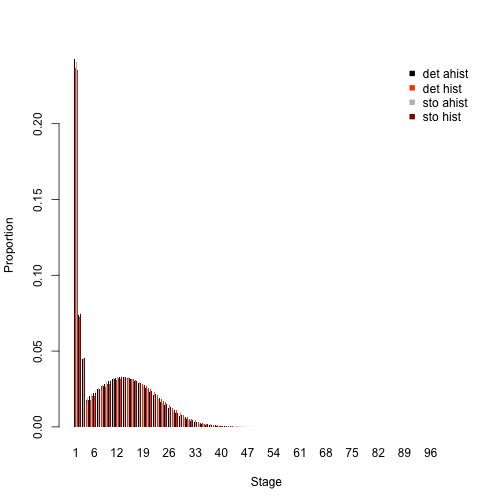Figure 6.5. Ahistorical vs. historically-corrected stable stage distribution par(lty = lty.o) Both ahistorical and historical approaches show the stable stage distribution dominated by the dormant seed stage. The next highest is the seedling stage, followed by the adult dormant stage. Stochastic analysis shows similar patterns. We will now move on to elasticity analysis. We will reduce the number of occasion steps simulated in the stochastic historical analysis to reduce the amount of computer processing time (this might take several hours even with the number of steps reduced). lath2ipmelas <- elasticity3(lath2ipmmean) lath3ipmelas <- elasticity3(lath3ipmmean) set.seed(42) lath2ipmelas_s <- elasticity3(lathmat2ipm, stochastic = TRUE) set.seed(42) lath3ipmelas_s <- elasticity3(lathmat3ipm, stochastic = TRUE, steps = 200) elas_put_together <- cbind.data.frame(colSums(lath2ipmelas$ah_elasmats[]),
Matrix::colSums(lath3ipmelas$ah_elasmats[]), colSums(lath2ipmelas_s$ah_elasmats[]),
Matrix::colSums(lath3ipmelas_s$ah_elasmats[])) names(elas_put_together) <- c("det ahist", "det hist", "sto ahist", "sto hist") rownames(elas_put_together) <- lath2ipmelas$ah_stages\$stage_id

lty.o <- par("lty")
par(lty = 0)
barplot(t(elas_put_together), beside=T, ylab = "Elasticity", xlab = "Stage",
col = c("black", "orangered", "grey", "darkred"), bty = "n")
legend("topright", c("det ahist", "det hist", "sto ahist", "sto hist"),
col = c("black", "orangered", "grey", "darkred"), pch = 15, bty = "n")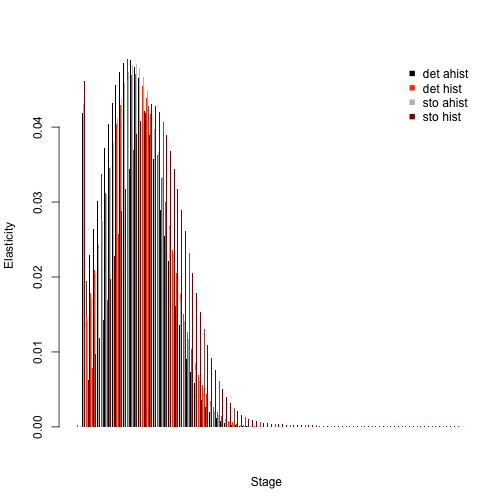Figure 6.6. Ahistorical vs. historically-corrected elasticity of lambda to stage
par(lty = lty.o)

The plot of these distributions shows the strong importance of vegetative dormancy and small adults. Both environmental stochasticity and individual history increase the elasticity associated with larger, though still small, adults.

Package lefko3 provides many other analytical capabilities. Please refer to the free e-manual called lefko3: a gentle introduction and other vignettes, all available on the projects page of the Shefferson lab website for more information.

## Acknowledgements

We are grateful to two anonymous reviewers whose scrutiny improved the quality of this vignette. The project resulting in this package and this tutorial was funded by Grant-In-Aid 19H03298 from the Japan Society for the Promotion of Science.

## Literature cited

Ehrlén, Johan. 2000. “The Dynamics of Plant Populations: Does the History of Individuals Matter?” Ecology 81 (6): 1675–84. https://doi.org/10.1890/0012-9658(2000)081[1675:TDOPPD]2.0.CO;2.